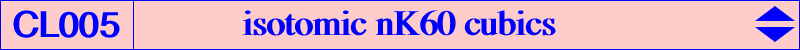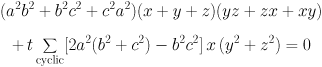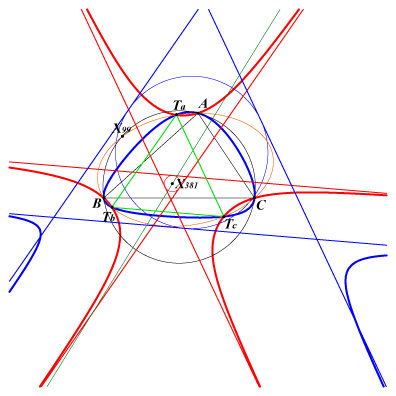All isotomic nK60 cubics have their root R on the line GX(76). They meet the Steiner circum-ellipse at A, B, C and three other points Ta, Tb, Tc which are the isotomic conjugates of the points at infinity of the sidelines of the Morley triangle. These points are the vertices of an equilateral triangle whose circumcircle is centered at X(76) and pass through X(99), the Steiner point. They have three real asymptotes parallel to the sidelines of this Morley triangle. Thus, they form a pencil of cubics generated by two special cases : when the root is G, the cubic decomposes into the line at infinity and the Steiner circum-ellipse. when the root is E = X(7757), the reflection of X(76) in G, the cubic is K093, the only nK0 of the pencil. If R is defined by vector(GR)= t vector(GE), the barycentric equation of the pencil is :The triangle formed by the asymptotes is always centered on the line passing through X(381) (midpoint of GH) and parallel to the Brocard line OK. This class of cubics contains K093 already mentioned, K089 (with singularity at G), K094 (a nK60+). When R = X(76), we find K198 : the triangle formed by the asymptotes is centered at X(381) and its circumradius is 2R. The figure below shows this cubic (blue curve) with K093 (red curve).